Showing first {{hits.length}} results of {{hits_total}} for {{searchQueryText}}{{hits.length}} results for {{searchQueryText}}

Contents 1 Integrals 2 Multiple integrals 3 Sums and products 4 Limits 5 Integer and sum limits improvement 6 Further reading Integrals Integral expression can be added using the \int_{lower}^{upper} command. Note, that integral expression may seems a little different in inline and display math mode - in inline mode the integral symbol and the limits are compressed. LaTeX code Output Integral $\int_{a}^{b} x^2 dx$ inside text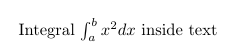$$\int_{a}^{b} x^2 dx$$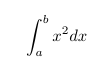Open an example in ShareLaTeX Multiple integrals To obtain double/triple/multiple integrals and cyclic integrals you must use amsmath and esint (for cyclic integrals) packages. LaTeX code Output $\iint_V \mu(u,v) \,du\,dv\iiint_V \mu(u,v,w) \,du\,dv\,dw\iiiint_V \mu(t,u,v,w) \,dt\,du\,dv\,dw\idotsint_V \mu(u_1,\dots,u_k) \,du_1 \dots du_k$$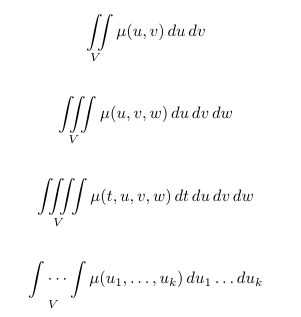$$\oint_V f(s) \,ds\oiint_V f(s,t) \,ds\,dt$$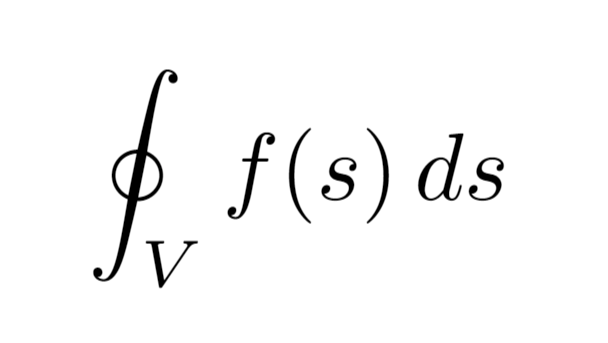Open an example in ShareLaTeX Sums and products Like integral, sum expression can be added using the \sum_{lower}^{upper} command. LaTeX code Output Sum \sum_{n=1}^{\infty} 2^{-n} = 1 inside text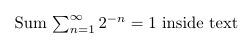$$\sum_{n=1}^{\infty} 2^{-n} = 1$$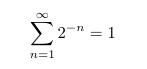In similar way you can obtain expression with product of a sequence of factors using the \prod_{lower}^{upper} command. LaTeX code Output Product \prod_{i=a}^{b} f(i) inside text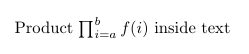$$\prod_{i=a}^{b} f(i)$$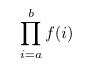Open an example in ShareLaTeX Limits Limit expression can be added using the \lim_{lower} command. LaTeX code Output Limit \lim_{x\to\infty} f(x) inside text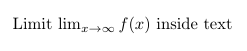$$\lim_{x\to\infty} f(x)$$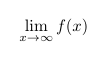Open an example in ShareLaTeX Integer and sum limits improvement In inline math mode the integral/sum/product lower and upper limits are placed right of integral symbol. Similar is for limit expressions. If you want the limits of an integral/sum/product to be specified above and below the symbol in inline math mode, use the \limits command before limits specification. LaTeX code Output Integral$\int_{a}^{b} x^2 dx$inside textImproved integral$\int\limits_{a}^{b} x^2 dx$inside text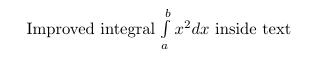Sum$\sum_{n=1}^{\infty} 2^{-n} = 1$inside textImproved sum$\sum\limits_{n=1}^{\infty} 2^{-n} = 1$inside text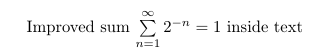Moreover, adding \displaystyle beforehand will make the symbol large and easier to read. On the other hand, \mathlarger command (provided by relsize package) is used to get bigger integral symbol in display.$\int\frac{1}{2}dx - \mathlarger{ \int\frac{1}{2}dx}\$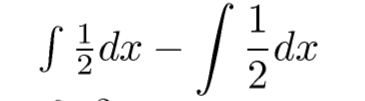Open an example in ShareLaTeX Further reading For more information see Mathematical expressions Subscripts and superscripts Fractions and Binomials Display style in math mode